Products - Herbs And SpicesAniseed
per 100g - R22.00
500g - R94.00
1kg - R184.00
3kg - R552.00
5kg - R921.00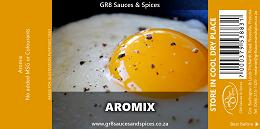Aromix
per 100g - R8.00
500g - R28.00
1kg - R52.00
3kg - R154.00
5kg - R257.00Basil
per 100g - R12.00
500g - R52.00
1kg - R98.00
3kg - R291.00
5kg - R488.00Bayleaves
per 100g - R20.00
500g - R88.00
1kg - R172.00
3kg - R516.00
5kg - R862.00Bicarbonate of Soda
per 100g - R7.00
500g - R14.00
1kg - R25.00
3kg - R72.00
5kg - R122.00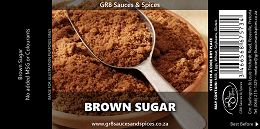Brown Sugar
2kg - R40.00
10kg - R195.00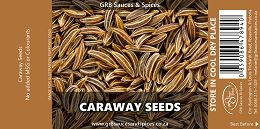Caraway Seeds
per 100g - R12.00
500g - R50.00
1kg - R99.00
3kg - R295.00
5kg - R490.00Celery Salt
per 100g - R8.00
500g - R28.00
1kg - R53.00
3kg - R156.00
5kg - R262.00Chilli - Coarse
per 100g - R12.00
500g - R48.00
1kg - R94.00
3kg - R279.00
5kg - R467.00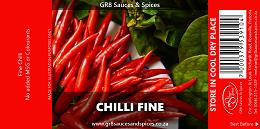Chilli - Fine
per 100g - R12.00
500g - R47.00
1kg - R93.00
3kg - R480.00
5kg - R455.00Cinnamon - Fine
per 100g - R15.00
500g - R64.00
1kg - R124.00
3kg - R370.00
5kg - R619.00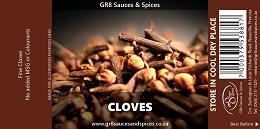Cloves - Fine
per 100g - R18.00
500g - R60.00
1kg - R115.00
3kg - R345.00
5kg - R570.00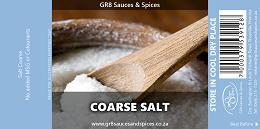Coarse Salt
per 100g - R3.00
500g - R3.00
1kg - R6.00
3kg - R17.00
5kg - R28.00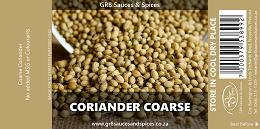Coriander Coarse
per 100g - R11.75
500g - R45.74
1kg - R87.48
3kg - R260.44
5kg - R435.40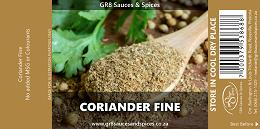Coriander Fine
per 100g - R11.21
500g - R43.04
1kg - R82.08
3kg - R244.24
5kg - R408.40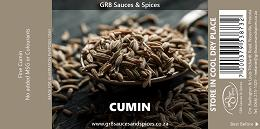Cumin
per 100g - R15.96
500g - R66.80
1kg - R129.60
3kg - R386.80
5kg - R646.00Fennel Coarse
per 100g - R10.67
500g - R40.34
1kg - R76.68
3kg - R228.04
5kg - R381.40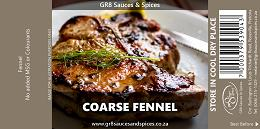Fennel Fine
per 100g - R15.42
500g - R64.10
1kg - R124.20
3kg - R370.60
5kg - R619.00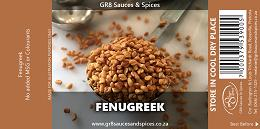Fenugreek
per 100g - R8.18
500g - R27.92
1kg - R51.84
3kg - R153.52
5kg - R257.20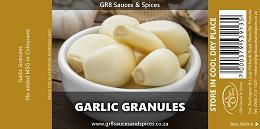Garlic - Granuals
per 100g - R16.82
500g - R71.12
1kg - R138.24
3kg - R412.72
5kg - R689.20Garlic And Herb
per 100g - R13.58
500g - R54.92
1kg - R105.84
3kg - R315.52
5kg - R527.20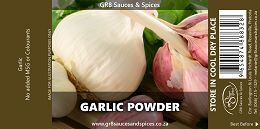Garlic Powder
per 100g - R16.82
500g - R71.12
1kg - R138.24
3kg - R412.72
5kg - R689.20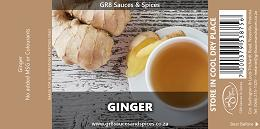Ginger
per 100g - R19.20
500g - R83.00
1kg - R162.00
3kg - R484.00
5kg - R808.00Gravy Powder
per 100g - R7.64
500g - R25.22
1kg - R46.44
3kg - R137.32
5kg - R230.20Himalayan Pink Salt
per 100g - R7.64
500g - R25.22
1kg - R46.44
3kg - R137.32
5kg - R230.20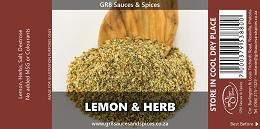Lemon & Herb
per 100g - R10.34
500g - R38.72
1kg - R73.44
3kg - R218.32
5kg - R365.20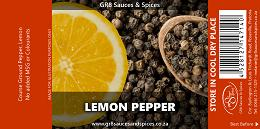Lemon Pepper
per 100g - R21.68
500g - R95.42
1kg - R186.84
3kg - R558.52
5kg - R932.20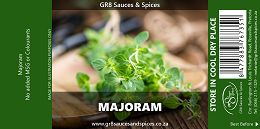Majoram
per 100g - R10.34
500g - R38.72
1kg - R73.44
3kg - R218.32
5kg - R365.20Meat Tenderizer
per 100g - R8.62
500g - R30.08
1kg - R56.16
3kg - R166.48
5kg - R278.80Mint
per 100g - R10.34
500g - R38.72
1kg - R73.44
3kg - R218.32
5kg - R365.20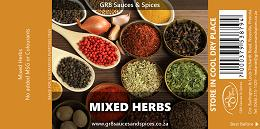Mixed herbs
per 100g - R17.80
500g - R75.98
1kg - R147.96
3kg - R441.88
5kg - R737.80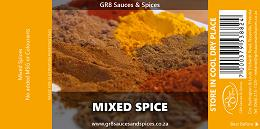Mixed Spice
per 100g - R22.01
500g - R97.04
1kg - R190.08
3kg - R568.24
5kg - R948.40Mustard Powder
per 100g - R15.53
500g - R64.64
1kg - R125.28
3kg - R373.84
5kg - R624.40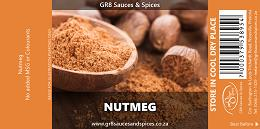Nutmeg
per 100g - R41.12
500g - R192.62
1kg - R381.24
3kg - R1,141.72
5kg - R1,904.20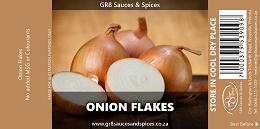Onion Flakes
per 100g - R11.42
500g - R44.12
1kg - R84.24
3kg - R250.72
5kg - R419.20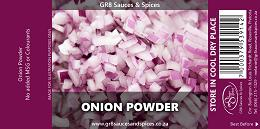Onion Powder
per 100g - R15.74
500g - R65.72
1kg - R127.44
3kg - R380.32
5kg - R635.20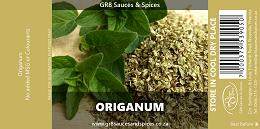Origanum
per 100g - R16.50
500g - R69.50
1kg - R135.00
3kg - R403.00
5kg - R673.00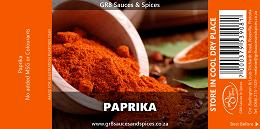Paprika
per 100g - R12.50
500g - R49.52
1kg - R95.04
3kg - R283.12
5kg - R473.20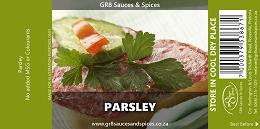Parsley
per 100g - R15.96
500g - R66.80
1kg - R129.60
3kg - R386.80
5kg - R646.00Pepper - Black coarse
per 100g - R21.68
500g - R95.42
1kg - R186.84
3kg - R558.52
5kg - R932.20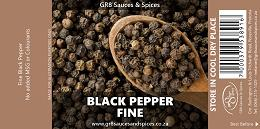Pepper - Black fine Select
per 100g - R20.60
500g - R90.02
1kg - R176.04
3kg - R526.12
5kg - R878.20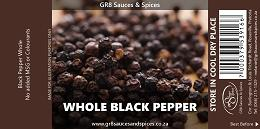Pepper - Black whole
per 100g - R19.52
500g - R84.62
1kg - R165.24
3kg - R493.72
5kg - R824.20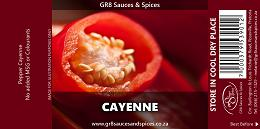Pepper - Cayenne
per 100g - R12.40
500g - R48.98
1kg - R93.96
3kg - R279.88
5kg - R467.80Pepper - White Select Fine
per 100g - R24.92
500g - R111.62
1kg - R219.24
3kg - R655.72
5kg - R1,094.20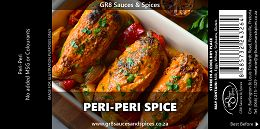Peri Peri
per 100g - R11.64
500g - R45.20
1kg - R86.40
3kg - R257.20
5kg - R430.00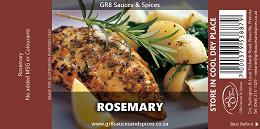Rosemary
per 100g - R18.44
500g - R79.22
1kg - R154.44
3kg - R461.32
5kg - R770.20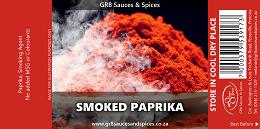Smoked Paprika
per 100g - R15.10
500g - R62.48
1kg - R120.96
3kg - R360.88
5kg - R602.80Smoked Salt
per 100g - R9.59
500g - R34.94
1kg - R65.88
3kg - R195.64
5kg - R327.40Thyme - Rubbed
per 100g - R15.96
500g - R66.80
1kg - R129.60
3kg - R386.80
5kg - R646.00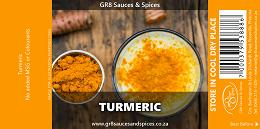Turmeric
per 100g - R11.96
500g - R46.82
1kg - R89.64
3kg - R266.92
5kg - R446.20